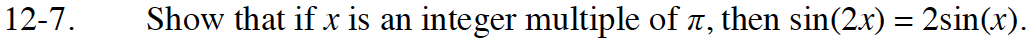### Home > INT3 > Chapter 12 > Lesson 12.1.1 > Problem12-7

12-7.

Show that if x is an integer multiple of π, then sin(2x) = 2sin(x). 12-7 HW eTool (Desmos)  Homework Help ✎Graph the equations y = sin (2x) and y = 2·sin(x) on graphing paper or use Desmos calculator.
Where are the two equations equal?

Use the Desmos calculator below to graph the equations.
Click the link at right for the full version of the Desmos calculator: Desmos